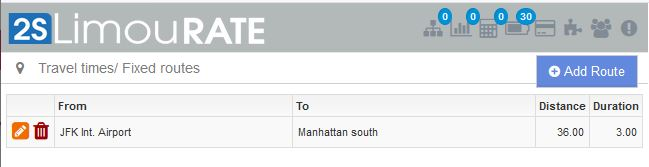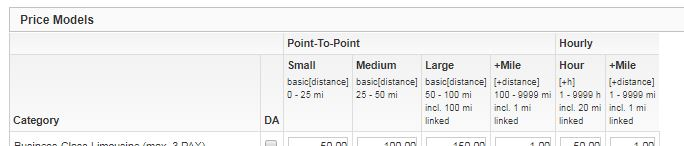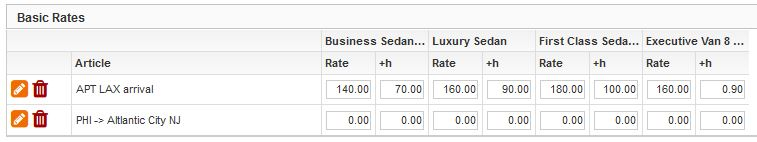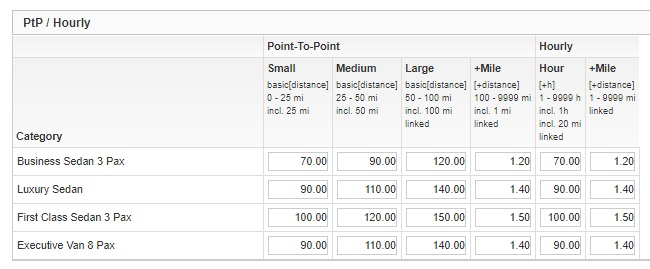## Calculation process

The calculation process runs through the following steps:

1. Travel time / Fixed route1.1 Check whether the trip between pickup and dropoff is covered by a fixed route. If this is the case, the time or distance stored on the fixed route is used as the basis for calculating the rate .
A calculation of the distance / duration of the trip via Google Maps does not take place in this case.1.2 The rate calculation is based on the values stored on the fixed routes according to the rules for PtP or hourly.

2. Basic RatesCheck whether the distance between pickup and dropoff is covered by a Basic Rate. If this is the case, the time or distance is not considered for the calculation. The rates deposited at the Basic Rates for each category are issued as the result of the calculation.

3. PtP / HourlyIf neither the Fixed routes nor the Basic Rates lead to a result, the rate is calculated based on the rules for Point-to-Point or Hourly.